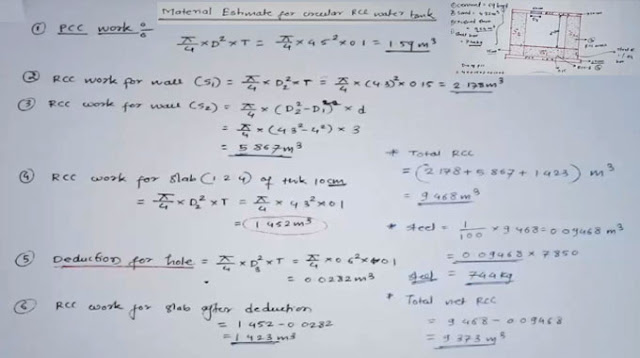Material Estimate of the RCC circular water tank

# Material Estimate of the RCC circular water tankDiagram representation for section/side view - RCC water tank is circular. Plain cement concrete = 1:2:4 with thickness = 10 cm. RCC = 1:2:4 with thickness = 10 cm. Opening for water tank is 60 cm with thickness = 10 cm. Thickness of RCC walls = 15 cm. 1% of 4M bars is used in wall. Depth of wall is 3 meter.

Diagram representation for top view - The diameter, D1, of center hole/circle is 4 m. Diameter of extra wall is 15 cm. Diameter of outer wall is 10 cm.
Top view diameter, D1, of center is the hole in section. 4.15 cm is up to the wall and 4.5 cm diameter is of PCC.

A - PCC work
Diameter of PCC = 4 + 0.15 * 2 + 0.10 * 2 = 4.5
meter π/4 * (Diameter of PCC)2 * Thickness of PCC = π/4 * (4.5)2 * 0.1 = 1.59 m3

B – RCC work for wall which is S1 π/4 * (D2)2 * Thickness = π/4 * 4.3 * 0.15 = 2.178 m3

C – RCC work for wall which is S2 π/4 * ((D2)2 – (D1)2) * Depth = π/4 *((4.3)2 – (4)2) * 3 = 5.867 m3

D - RCC work for slab (1:2:4) of thickness 10 cm = π/4 * (D2)2 * Thickness = π/4 * (4.3)2 * 0.1 = 1.452 m3

E – Deduction for hole π/4 * (D3)2 * Thickness = π/4 * (0.60)2 * 0.1 = 0.0282 m3 F – RCC Work RCC work for slab after deduction = 1.452 – 0.0282 = 1.423 m3

G – Total RCC (2.178 + 5.867 + 1.423) m3 = 9.468 m3
* Steel = 1/100 * 9.468 = 0.09468 m3 = 0.09468 * 7850 = 744 kg = required steel

-Total net RCC = 9.468 – 0.09468 = 9.373 m3
-Total cement, sand, crushed stone volume = 9.373 + 1.59 = 10.963 m3
H – Ratio Cement: Sand:
Crush Aggregate/stone
Material = Ratio of material/Sum of ratio * Dry Volume
Cement = C/C+S+CA * Dry Volume
Sand = S/C+S+CA * Dry Volume
Total concrete work = 10.963 m3
Ratio = 1:2:4
Sum of ratio = 7
Dry Volume = Wet Volume * 1.54
10.963 * 1.54 = 16.883 m3
Materials = Ratio of materials/sum of material * Dry Volume
Cement = 1/7 * 16.883 = 2.41 m3

Reference: constructioncivilengineering.com

Continue Reading about the "Material Estimate of the RCC circular water tank" on the next page below